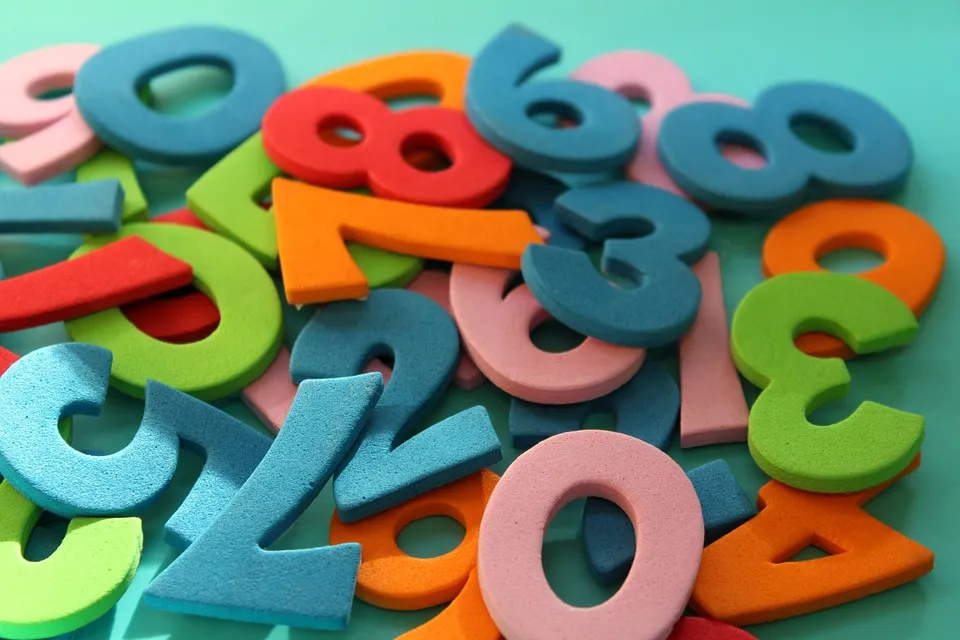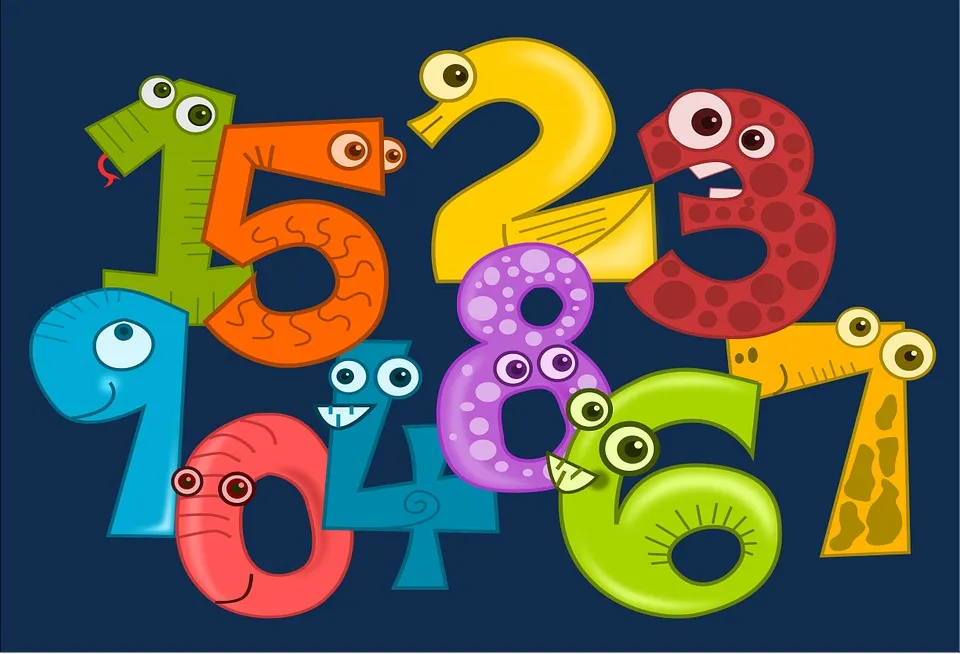# Divisibility Rule For 11

Hi there. In this short math post, I cover the divisibility rule for 11. This math topic falls under the math branch of number theory. As number theory is not my main math specialty, I would not know of real life applications for this topic.Pixabay Image Source

## Topics

• Multiples Of Eleven
• The Divisibility Rule For 11

## Multiples Of Eleven

I think it is a good idea to cover the multiples of eleven before getting into the divisibility rule for eleven. A multiple is the result of a number multiplied by a whole number. Three is a multiple of three as we have `3 x 1 = 3`. Six is also a multiple of 3 as we have `3 x 2 = 6`. Other multiples of 3 include 9, 12, 15, 18, 21 and so on.

For the multiples of eleven we have 11, 22, 33, 44, 55, 66 and so on. I have included a table below for the first couple of multiples for 11.

Multiplication ExpressionMultiple
11 x 111
11 x 222
11 x 333
11 x 444
11 x 555
11 x 666
11 x 777
11 x 888
11 x 999
11 x 10110
11 x 11121
11 x 12132Pixabay Image Source

## The Divisibility Rule For 11

The divisibility rule for 11 is a procedure that is repeated until a stop condition. Here is the rule.

1. Given a large number, take the last digit.
2. Use the rest of the digits minus this last digit.
3. If the answer from subtraction is a multiple of 11 then that large number is divisible by 11. If the answer is not a multiple of 11, then the original number is not divisible by 11. Restart on step 1 if you are not sure.

Example One

Is 1234 divisible by 11?

The last digit here is 4. Use this 4 to subtract from 123. This would be `123 - 4 = 119`. The number 119 is not divisible by 11 so 1234 is not divisible by 11. (You could do 11 minus 9 to confirm.)

Example Two

Check if 2717 is divisible by 11.

Use the last digit of 7 and have 271 subtracted by 7. The answer from 271 minus 7 is 264. This number is fairly large to determine if it is a multiple of 11. Run the procedure again.

From 264 use 26 minus 4 to get 22. Twenty-two is a multiple of 11 which makes 2717 divisible by 11.

Example Three

Is 77777 divisible by 11?

To start do 7777 minus 7 to obtain 7770. The next step is to repeat the procedure. The last digit is 0 which would make the subtraction 777 minus 0 which is just 777. The procedure is repeated again. Subtract 77 and 7 to get 70. Seventy is not divisible by 11 so 77777 is not divisible by 11.Pixabay Image Source